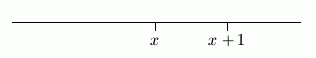﻿ Identifying Inequalities with Variables as True or False
IDENTIFYING INEQUALITIES WITH VARIABLES AS TRUE OR FALSE
by Dr. Carol JVF Burns (website creator)
Follow along with the highlighted text while you listen!
• PRACTICE (online exercises and printable worksheets)
Want more details, more exercises?

An Inequality that is Always True

Consider the inequality ‘$\,x < x + 1\,$’.
Let $\,x\,$ be any real number.
Then, $\,x+1\,$ lies one unit to the right of $\,x\,$ on a number line.
Therefore, $\,x\,$ always lies to the left of $\,x+1\,$,
so the sentence ‘$\,x < x + 1\,$’ is always true.An Inequality that is Always False

Consider the inequality ‘$\,x -1> x\,$’.
Let $\,x\,$ be any real number.
Then, $\,x-1\,$ lies one unit to the left of $\,x\,$ on a number line.
Therefore, $\,x-1\,$ never lies to the right of $\,x\,$,
so the sentence ‘$\,x-1 > x\,$’ is always false.For these exercises, you should think in terms of position on a number line,
as in the previous examples.

EXAMPLES:

Determine if each inequality is ALWAYS TRUE or ALWAYS FALSE.

‘$\,x-1 < x + 1\,$’     is     Always True
‘$\,x > x + 2\,$’     is     Always False
Master the ideas from this section
Solving Equations of the Form $\,xy = 0$